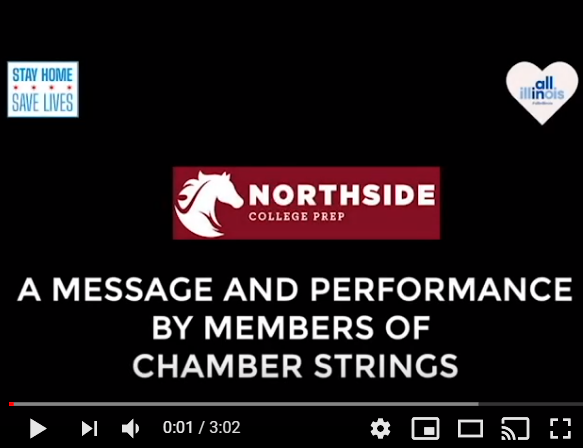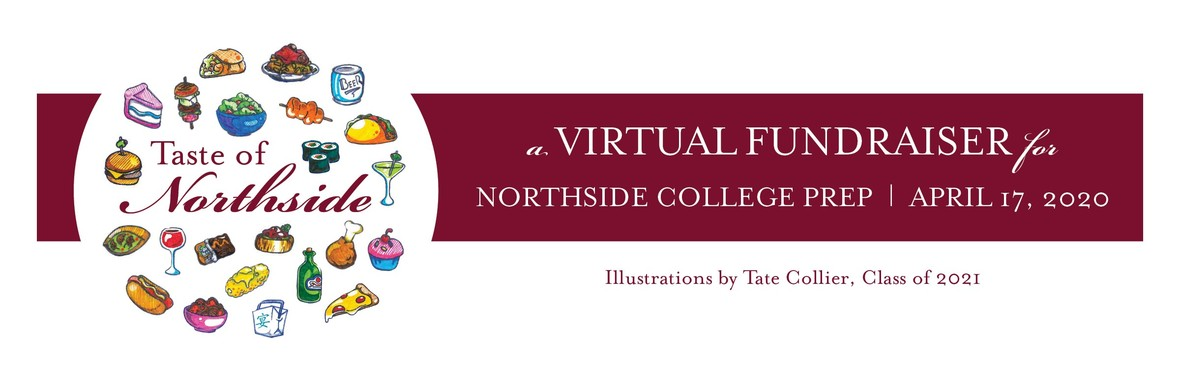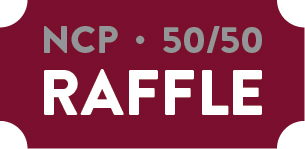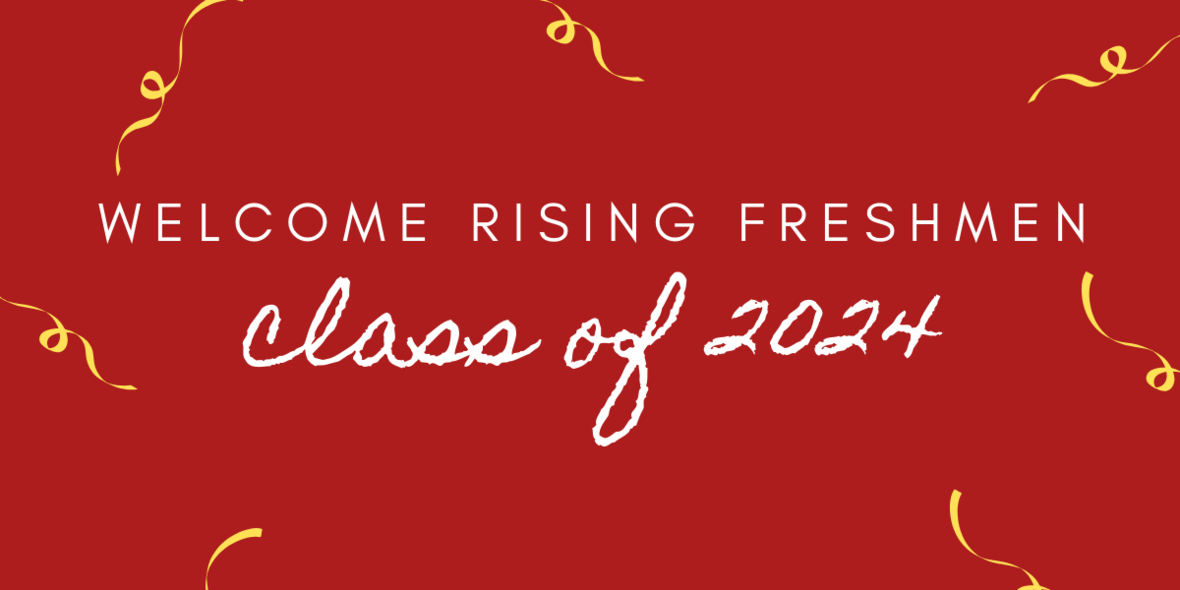# A Message from CPS:

While we know this is not the spring break you anticipated, we hope this week has provided you a chance to relax and gear up for remote learning, which begins this Monday, April 13.

CPS is committed to providing high-quality learning options for all students from Monday, April 13, through the end of our state-mandated closure. Please note that remote learning will not take place on Friday, April 17, as it is a professional development day for teachers on the CPS calendar.

We urge all families to visit cps.edu/remotelearning for the most up-to-date information on remote learning and to access valuable remote learning resources for their children. For students participating in remote learning virtually, please see these best practices for important information about privacy and confidentiality.

On Monday, we will all begin a journey into uncharted territory. It’s natural to feel some anxiety, but we know that if we work together, we can provide our children with the education they need to remain on track and continue growing until we can all be together again. We thank you for your partnership and look forward to starting this new chapter as one CPS family.

 table div table+table+table div table{width:100%;padding:0}table div table+table+table div table img{width:96.23%;padding:0;float:none}table div table+table+table div table td{width:100%;padding:0 1.88% 18px}/* styles */# A Message and Performance from NCP's Chamber StringsClick the image for a message and a performance by members of NCP's Chamber Strings.
 table div table+table+table+table+table+table div table{width:100%;padding:0}table div table+table+table+table+table+table div table img{width:96.23%;padding:0;float:none}table div table+table+table+table+table+table div table td{width:100%;padding:0 1.88% 18px}/* styles */# Please Continue to Support the Paddle Raise to Build a New Turf Field

This year’s Paddle Raise is dedicated to raising funds for a much-needed, new turf athletic field. Every year, more gym classes and home athletic games must be cancelled because our Northside field is in such poor condition. Heavy use over the years has rendered it virtually unusable. A new turf field will allow our students, and future Northsiders, to play and exercise outside safely throughout the year. Let's give all of them a home-field advantage. Please demonstrate your strong support for this long-overdue improvement to the Northside campus by making a monetary contribution to the 2020 Paddle Raise.

Participate in the Paddle Raise for NCP's new turf field. Our Northside video club, the Mustang Reel, created THIS VIDEO to inspire your generosity. Let's all PONY UP, and then click HERE to see the impact of your contribution.

# Virtual "Live Auction" Postponed Indefinitely

As you know, due to the COVID-19 pandemic, we converted the Taste of Northside Spring Benefit into a virtual event, which we intended to host this Friday, April 17. Given that so much is still in flux--including the economy and whether the offered sporting events and concert will take place this season--we feel we must postpone the virtual event to a later (to be determined) date when things stabilize. We hope, for countless reasons, that will be sooner rather than later. Until then, we will sit tight and assess the evolving situation. Look for future announcements regarding the virtual event here, in the eblast, and on Facebook. As always, we sincerely thank you for your continued support and patience.# Raffle Drawing Still On

The 50/50 Raffle drawing will take place, as planned, this Friday, April 17, at 10 p.m. The winner will be chosen by our auction platform's automated, blind system. The winner will be contacted on Saturday, April 18, and announced in the eblast on Sunday, April 19, provided the winner approves of the disclosure.

The Raffle Pool is up to \$3,750.00. Help us reach this year's goal of \$10,000 in raffle sales! The drawing is on April 17. Click HERE to buy one chance for \$25 and HERE to purchase five chances for \$100.

Buy tickets now to increase your chances of winning and to raise critical funds for Northside!

# Pick Up of Silent-Auction Items

Thank you to those of you who have contacted Kristen Werries Collier to coordinate picking up the silent-auction items that you won. If you have not done so, please email her at kristenwerriescollier@gmail.com. Pick ups are taking place in a safe, social-distancing manner from the front porch of her Wicker-Park home. For those of you who do not want to venture out, Kristen will continue to hold your items and future arrangements will be made.

 /* styles */ To pay any remaining open balance, please go to Northside.GiveSmart.com. Thank you for your support!
 /* styles */ Questions? Email Debra Swan, Event Chair at debra@theswans.net.
 table div table+table+table+table+table+table+table+table+table+table+table+table+table+table+table+table+table div table{width:100%;padding:0}table div table+table+table+table+table+table+table+table+table+table+table+table+table+table+table+table+table div table img{width:96.23%;padding:0;float:none}table div table+table+table+table+table+table+table+table+table+table+table+table+table+table+table+table+table div table td{width:100%;padding:0 1.88% 18px}/* styles */# One Summer Chicago Application Is Open!

## Summer Jobs Available for Students - Apply Online by May 20

One Summer Chicago brings together government institutions, community-based organizations, and companies to offer over 30,000 employment and internship opportunities
to youth and young adults ages 14 to 24.

Students can apply HERE.

 table div table+table+table+table+table+table+table+table+table+table+table+table+table+table+table+table+table+table+table+table div table{width:100%;padding:0}table div table+table+table+table+table+table+table+table+table+table+table+table+table+table+table+table+table+table+table+table div table img{width:96.23%;padding:0;float:none}table div table+table+table+table+table+table+table+table+table+table+table+table+table+table+table+table+table+table+table+table div table td{width:100%;padding:0 1.88% 18px}/* styles *//* styles */ Follow Northside on Instagram: https://www.instagram.com/northsidecollegeprep/ Follow Northside's Asian club on Instagram: https://www.instagram.com/ncpaasa/
 table div table+table+table+table+table+table+table+table+table+table+table+table+table+table+table+table+table+table+table+table+table+table+table+table div table{width:100%;padding:0}table div table+table+table+table+table+table+table+table+table+table+table+table+table+table+table+table+table+table+table+table+table+table+table+table div table img{width:96.23%;padding:0;float:none}table div table+table+table+table+table+table+table+table+table+table+table+table+table+table+table+table+table+table+table+table+table+table+table+table div table td{width:100%;padding:0 1.88% 18px}/* styles */## Remote Learning begins Monday, April 13

Read the NCP Communication from Saturday, April 04 HERE.

Read the NCP Communication from Monday, March 30 HERE.

 table div table+table+table+table+table+table+table+table+table+table+table+table+table+table+table+table+table+table+table+table+table+table+table+table+table+table+table div table{width:100%;padding:0}table div table+table+table+table+table+table+table+table+table+table+table+table+table+table+table+table+table+table+table+table+table+table+table+table+table+table+table div table img{width:96.23%;padding:0;float:none}table div table+table+table+table+table+table+table+table+table+table+table+table+table+table+table+table+table+table+table+table+table+table+table+table+table+table+table div table td{width:100%;padding:0 1.88% 18px}/* styles */# Parent Questions - For Parents Only

As a reminder, this Question Form is available to submit your questions to the NCP administration. Please note that you will not receive a direct response to your question. Instead, this form will be used as a repository for parent questions. Once the admin team receives information or finds answers to these questions, they will be sent out as part of the parent updates.

 table div table+table+table+table+table+table+table+table+table+table+table+table+table+table+table+table+table+table+table+table+table+table+table+table+table+table+table+table+table div table{width:100%;padding:0}table div table+table+table+table+table+table+table+table+table+table+table+table+table+table+table+table+table+table+table+table+table+table+table+table+table+table+table+table+table div table img{width:96.23%;padding:0;float:none}table div table+table+table+table+table+table+table+table+table+table+table+table+table+table+table+table+table+table+table+table+table+table+table+table+table+table+table+table+table div table td{width:100%;padding:0 1.88% 18px}/* styles */# NCP Senior's Artwork Featured in Sun-Times Article

Congratulations to NCP senior Tarik Brown, who was interviewed by the Chicago Sun-Times this week. The article, titled, Coronavirus drives Chicago artists,
outlined how the pandemic is showing up in all sorts of ways in the work of Chicago artists. It also features an image of his work and discusses the motivation behind his artwork during the pandemic.

 table div table+table+table+table+table+table+table+table+table+table+table+table+table+table+table+table+table+table+table+table+table+table+table+table+table+table+table+table+table+table+table+table div table{width:100%;padding:0}table div table+table+table+table+table+table+table+table+table+table+table+table+table+table+table+table+table+table+table+table+table+table+table+table+table+table+table+table+table+table+table+table div table img{width:96.23%;padding:0;float:none}table div table+table+table+table+table+table+table+table+table+table+table+table+table+table+table+table+table+table+table+table+table+table+table+table+table+table+table+table+table+table+table+table div table td{width:100%;padding:0 1.88% 18px}/* styles */# Parents & Friends of the Class of 2020 - Ad Space in Yearbook Available

To commemorate your student's accomplishments or declare your best wishes, The Stampede, Northside's yearbook, offers ad space in its upcoming edition. The prices are as follows: full page \$150.00; half page \$80; quarter page \$50. Please see the order form for details:

All materials are due by Monday, April 13.

 table div table+table+table+table+table+table+table+table+table+table+table+table+table+table+table+table+table+table+table+table+table+table+table+table+table+table+table+table+table+table+table+table+table+table+table div table{width:100%;padding:0}table div table+table+table+table+table+table+table+table+table+table+table+table+table+table+table+table+table+table+table+table+table+table+table+table+table+table+table+table+table+table+table+table+table+table+table div table img{width:96.23%;padding:0;float:none}table div table+table+table+table+table+table+table+table+table+table+table+table+table+table+table+table+table+table+table+table+table+table+table+table+table+table+table+table+table+table+table+table+table+table+table div table td{width:100%;padding:0 1.88% 18px}/* styles */# The Stampede is Collecting Quarantine Pics, Artwork, and Quotes

Due to the impact that the novel coronavirus has had on the 2019-2020 school year, the Stampede is dedicating several pages of the yearbook to documenting students' experiences during quarantine.

Please encourage your students to access the form and answer any of the questions. The Stampede is also asking students to submit any photos, artwork, poetry, etc. from quarantine that they would be comfortable appearing in the yearbook. The students will be receiving a link to the form in their Monday announcements. Thank you and stay safe!

 table div table+table+table+table+table+table+table+table+table+table+table+table+table+table+table+table+table+table+table+table+table+table+table+table+table+table+table+table+table+table+table+table+table+table+table+table+table div table{width:100%;padding:0}table div table+table+table+table+table+table+table+table+table+table+table+table+table+table+table+table+table+table+table+table+table+table+table+table+table+table+table+table+table+table+table+table+table+table+table+table+table div table img{width:96.23%;padding:0;float:none}table div table+table+table+table+table+table+table+table+table+table+table+table+table+table+table+table+table+table+table+table+table+table+table+table+table+table+table+table+table+table+table+table+table+table+table+table+table div table td{width:100%;padding:0 1.88% 18px}/* styles */# It's Not Too Late to Purchase Your 2019-2020 Yearbook

Yearbooks are still on sale for \$55:

1. Go to tinyurl.com/stampede2020
2. Enter the code: 4187220
3. Purchase!
 1 Go to tinyurl.com/stampede2020
 2 Enter the code: 4187220
 3 Purchase!
 table div table+table+table+table+table+table+table+table+table+table+table+table+table+table+table+table+table+table+table+table+table+table+table+table+table+table+table+table+table+table+table+table+table+table+table+table+table+table+table div table{width:100%;padding:0}table div table+table+table+table+table+table+table+table+table+table+table+table+table+table+table+table+table+table+table+table+table+table+table+table+table+table+table+table+table+table+table+table+table+table+table+table+table+table+table div table img{width:96.23%;padding:0;float:none}table div table+table+table+table+table+table+table+table+table+table+table+table+table+table+table+table+table+table+table+table+table+table+table+table+table+table+table+table+table+table+table+table+table+table+table+table+table+table+table div table td{width:100%;padding:0 1.88% 18px}/* styles */## Northside Track Teams Workout Together at Home

The Men's and Women's track teams have been using Zoom meetings to link up and do daily core workouts and bodyweight circuits. Essentially, these are group workouts, but they count reps together and seem to be having a lot of fun connecting at the same time.

 table div table+table+table+table+table+table+table+table+table+table+table+table+table+table+table+table+table+table+table+table+table+table+table+table+table+table+table+table+table+table+table+table+table+table+table+table+table+table+table+table+table div table{width:100%;padding:0}table div table+table+table+table+table+table+table+table+table+table+table+table+table+table+table+table+table+table+table+table+table+table+table+table+table+table+table+table+table+table+table+table+table+table+table+table+table+table+table+table+table div table img{width:96.23%;padding:0;float:none}table div table+table+table+table+table+table+table+table+table+table+table+table+table+table+table+table+table+table+table+table+table+table+table+table+table+table+table+table+table+table+table+table+table+table+table+table+table+table+table+table+table div table td{width:100%;padding:0 1.88% 18px}/* styles */# A Big Welcome to Parents of the 2024 NCP Freshmen!

 table div table+table+table+table+table+table+table+table+table+table+table+table+table+table+table+table+table+table+table+table+table+table+table+table+table+table+table+table+table+table+table+table+table+table+table+table+table+table+table+table+table+table+table+table div table{width:100%;padding:0}table div table+table+table+table+table+table+table+table+table+table+table+table+table+table+table+table+table+table+table+table+table+table+table+table+table+table+table+table+table+table+table+table+table+table+table+table+table+table+table+table+table+table+table+table div table img{width:96.23%;padding:0;float:none}table div table+table+table+table+table+table+table+table+table+table+table+table+table+table+table+table+table+table+table+table+table+table+table+table+table+table+table+table+table+table+table+table+table+table+table+table+table+table+table+table+table+table+table+table div table td{width:100%;padding:0 1.88% 18px}/* styles */All these great restaurants and food and beverage providers stepped up to donate their offerings for the Benefit. Many are in our own backyard and we’d like to help see them be here, serving our communities, when our city is fully operational again. Some are operating with online orders and curbside pickup. Others are closed for the next two weeks. Please consider ordering from them or purchase a gift card for a later use. Say 'thank you from the Northside community'.

 table div table+table+table+table+table+table+table+table+table+table+table+table+table+table+table+table+table+table+table+table+table+table+table+table+table+table+table+table+table+table+table+table+table+table+table+table+table+table+table+table+table+table+table+table+table+table+table div table{width:100%;padding:0}table div table+table+table+table+table+table+table+table+table+table+table+table+table+table+table+table+table+table+table+table+table+table+table+table+table+table+table+table+table+table+table+table+table+table+table+table+table+table+table+table+table+table+table+table+table+table+table div table img{width:96.23%;padding:0;float:none}table div table+table+table+table+table+table+table+table+table+table+table+table+table+table+table+table+table+table+table+table+table+table+table+table+table+table+table+table+table+table+table+table+table+table+table+table+table+table+table+table+table+table+table+table+table+table+table div table td{width:100%;padding:0 1.88% 18px}/* styles */## FROM THE COUNSELING DEPARTMENT

 /* styles */ Updates from the Counseling Department • Freshmen • Sophomores • Juniors • Seniors • Senior Spring Checklist
 table div table+table+table+table+table+table+table+table+table+table+table+table+table+table+table+table+table+table+table+table+table+table+table+table+table+table+table+table+table+table+table+table+table+table+table+table+table+table+table+table+table+table+table+table+table+table+table+table+table+table div table{width:100%;padding:0}table div table+table+table+table+table+table+table+table+table+table+table+table+table+table+table+table+table+table+table+table+table+table+table+table+table+table+table+table+table+table+table+table+table+table+table+table+table+table+table+table+table+table+table+table+table+table+table+table+table+table div table img{width:96.23%;padding:0;float:none}table div table+table+table+table+table+table+table+table+table+table+table+table+table+table+table+table+table+table+table+table+table+table+table+table+table+table+table+table+table+table+table+table+table+table+table+table+table+table+table+table+table+table+table+table+table+table+table+table+table+table div table td{width:100%;padding:0 1.88% 18px}/* styles */table div table+table+table+table+table+table+table+table+table+table+table+table+table+table+table+table+table+table+table+table+table+table+table+table+table+table+table+table+table+table+table+table+table+table+table+table+table+table+table+table+table+table+table+table+table+table+table+table+table+table+table+table div table{width:100%;padding:0}table div table+table+table+table+table+table+table+table+table+table+table+table+table+table+table+table+table+table+table+table+table+table+table+table+table+table+table+table+table+table+table+table+table+table+table+table+table+table+table+table+table+table+table+table+table+table+table+table+table+table+table+table div table img{width:96.23%;padding:0;float:none}table div table+table+table+table+table+table+table+table+table+table+table+table+table+table+table+table+table+table+table+table+table+table+table+table+table+table+table+table+table+table+table+table+table+table+table+table+table+table+table+table+table+table+table+table+table+table+table+table+table+table+table+table div table td{width:100%;padding:0 1.88% 18px}/* styles */